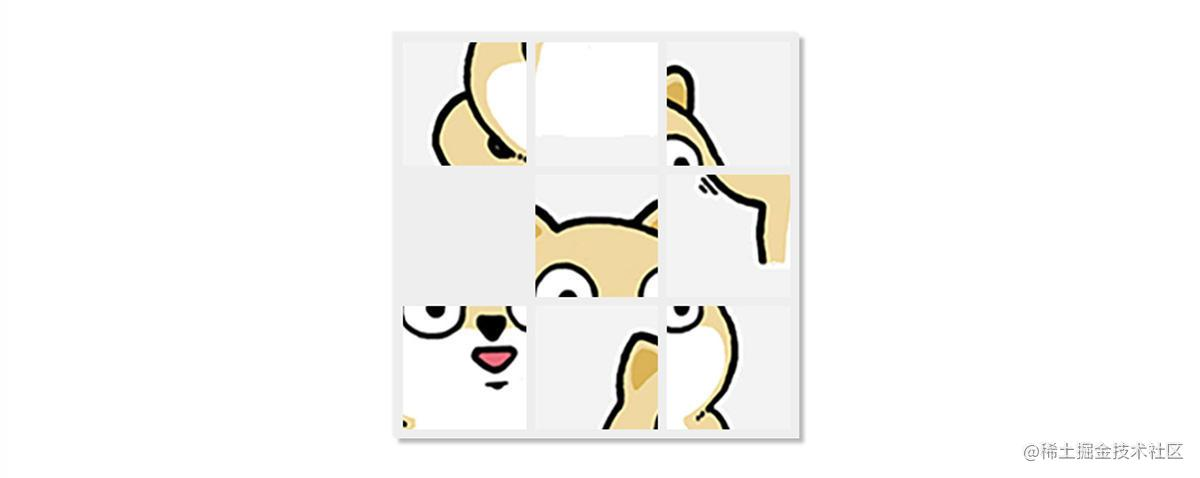# 用 JavaScript 实现简单拼图游戏本篇主要讲解，如何利用原生的 `JavaScript` 来实现一个简单的拼图小游戏。

# 一、游戏的基础逻辑

### 1、图形绘制

``````<canvas id="background" width="450px" height="450px"></canvas>
``````
``````var background = document.getElementById("background");
var context = background.getContext('2d');
``````

``````var drawImageItem = function(index, position) {
var img = new Image();
img.src = './image/dog_0' + String(index+1) + '.jpg';
var rect = rectForPosition(position);
context.drawImage(img, rect, rect, rect, rect);
}
}
``````

``````var originRect = rectForPosition(origin);
context.clearRect(originRect, originRect, originRect, originRect);
``````

### 2、事件处理

`JavaScript` 中对触屏和鼠标点击的监听是一样的，都是通过 `canvas``onclick` 事件进行回调，具体如下：

``````// 屏幕点击
background.onclick = function(e) {
};
``````

``````if (event.keyCode == '37') {  // 左
// do something
} else if (event.keyCode == '38') { // 上
// do something
} else if (event.keyCode == '39') { // 右
// do something
} else if (event.keyCode == '40') { // 下
// do something
}
``````

### 3、定时器

``````setInterval("run()", 100);
``````

# 二、拼图的基础逻辑

### 1、生成随机序列

``````var setupRandomPosition = function() {
var list1 = [4, 3, 2, 8, 0, 7, 5, 6, 1];
var list2 = [2, 0, 5, 6, 8, 7, 3, 1, 4];
var list3 = [3, 7, 2, 4, 1, 6, 8, 0, 5];
var list4 = [3, 2, 4, 1, 7, 6, 5, 0, 8];
var lists = [list1, list2, list3, list4];

imageIndexForPosition = lists[parseInt(Math.random() * 4)];

// 获取空位位置
var emptyPosition = 0;
for (var i = imageIndexForPosition.length - 1; i >= 0; i--) {
if (imageIndexForPosition[i] == lastIndex()) {
emptyPosition = i;
break;
}
}
background.emptyPosition = emptyPosition;

// 随机移动次数
var times = 10;
while (times--) {
// 获取随机数，决定空位哪个位置进行移动
var direction = parseInt(Math.random() * 4);

var target = -1;
if (direction == 0) {
target = topOfPosition(emptyPosition);  // 上
} else if (direction == 1) {
target = leftOfPosition(emptyPosition);  // 左
} else if (direction == 2) {
target = rightOfPosition(emptyPosition);  // 右
} else if (direction == 3) {
target = bottomOfPosition(emptyPosition);  // 下
}
if (target < 0 || target > lastIndex()) {  // 位置不合法，继续下一次循环
continue;
}
var result = moveImageIfCanAtPosition(target);
if (result >= 0) { // 如果移动成功，更新空位的位置
emptyPosition = target;
}
}
}
``````

### 2、判断是否可以移动方块

``````var isPositionEmpty = function(position) {
if (position < 0 || position > lastIndex()) {
return false;
}
if (imageIndexForPosition[position] == lastIndex()) {
return true;
} else {
return false;
}
}
``````

### 3、实现方块移动

``````var refreshImagePositions = function(origin, target) {
var originRect = rectForPosition(origin);
context.clearRect(originRect, originRect, originRect, originRect);
drawImageItem(imageIndexForPosition[target], target);
}
``````

### 4、检查是否完成

``````var checkIfFinish = function() {
for (var index = 0; index < imageIndexForPosition.length; index++) {
if (index != imageIndexForPosition[index]) {
return false;
}
}
return true;
}
``````

### 5、交互事件屏蔽

``````// 屏幕点击
background.onclick = function(e) {
if (isFinish) {
return;
}

// do something
};

// 键盘按钮事件
document.onkeyup = function(event) {
if (isFinish) {
return;
}

// do something
}
``````

# 参考

JavaScript 教程

• Chokcoco
9月前• 桃小瑞
5月前••••• ssssyoki
5年前
• C92
5年前
• wuhou123
1年前••• JYeontu
10月前• CUGGZ
2年前